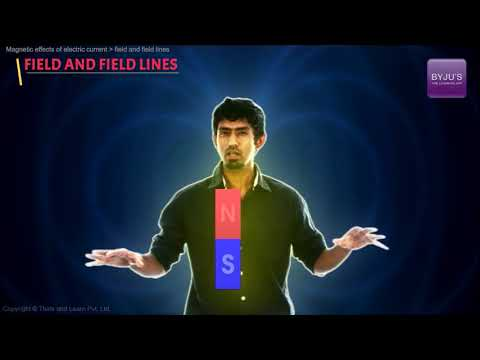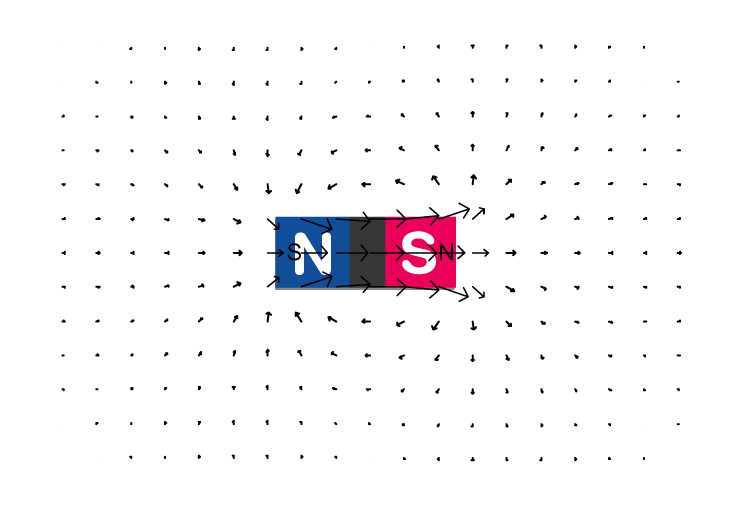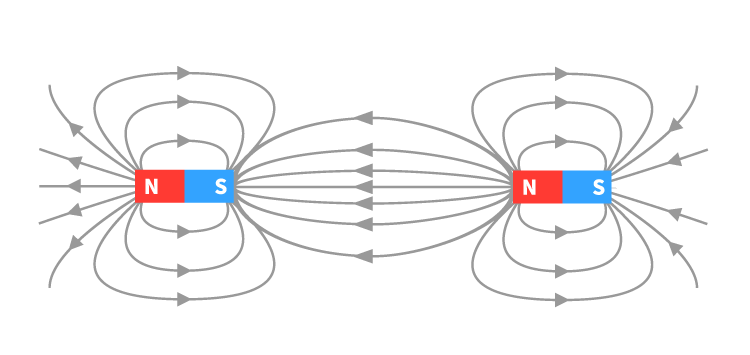# Magnetic Field

A magnetic field is a vector field in the neighbourhood of a magnet, electric current, or changing electric field, in which magnetic forces are observable.  A magnetic field is produced by moving electric charges and intrinsic magnetic moments of elementary particles associated with a fundamental quantum property known as the spin.  Magnetic field and electric field are both interrelated to each other and are components of the electromagnetic force, one of the four fundamental forces of nature.

## History

• The research on the magnetic field began in 1269 when French scholar Petrus Peregrinus de Maricourt mapped out the magnetic field on the surface of a spherical magnet using iron needles. He noticed that the resulting field lines crossed at two points. He named these points “poles.” After this observation, he stated that magnets always have North and South pole irrespective of how finely one slices them.
• Three centuries later, William Gilbert stated that Earth is a magnet.
• John Mitchell, an English clergyman, and philosopher, in 1750 stated that magnetic poles attract and repel each other.
• In 1785, Charles-Augustin de Coulomb experimentally verified Earths’ magnetic field. Followed by this, in the 19th century, French mathematician and geometer Simeon Denis Poisson created the first model of the magnetic field, which he presented in 1824.
• By the 19th century, further revelations refined and challenged previously-held notions.
• In 1819, Danish physicist and chemist Hans Christian Orsted discovered that an electric current creates a magnetic field around it.
• In 1825, André-Marie Ampère proposed a model of magnetism where this force was due to perpetually flowing loops of current, instead of the dipoles of magnetic charge.
• In 1831, English scientist Faraday showed that a changing magnetic field generates an electric field. In effect, he discovered electromagnetic induction.
• Between 1861 and 1865, James Clerk Maxwell published theories on electricity and magnetism. It was known as Maxwell’s equation. These equations described the relationship between electricity and magnetism.

## What is a Magnetic Field?

Magnetic Field is the region around a magnetic material or a moving electric charge within which the force of magnetism acts.A pictorial representation of the magnetic field which describes how a magnetic force is distributed within and around a magnetic material

 Symbol B or H Unit Telsa Base Unit (Newton.Second)/Coulomb

### Video Explaning Magnetic Field## Illustration of Magnetic Field

Typically, a magnetic field can be illustrated in two different ways.

• Magnetic Field Vector
• Magnetic Field Lines

### Magnetic Field Vector

The magnetic field can be mathematically described as a vector field. The vector field is a set of many vectors that are drawn on a grid. In this case, each vector points in the direction that a compass would point and has a length that is dependent on the strength of the magnetic force.Vector Field of a Bar Magnet

### Magnetic Field Lines

The use of field lines is an alternative way to represent the information contained within a magnetic vector field. Magnetic field lines are imaginary lines.

Magnetic field lines are a visual tool used to represent magnetic fields. They describe the direction of the magnetic force on a north monopole at any given position

The density of the lines indicates the magnitude of the field. Taking an instance, the magnetic field is stronger and crowded near the poles of a magnet. As we move away from the poles it is weak and the lines become less dense.The figure shows a magnetic field lines plot for a bar magnet

#### Properties of Magnetic Field Lines

• Magnetic field lines never cross each other
• The density of the field lines indicates the strength of the field
• Magnetic field lines always make closed-loops
• Magnetic field lines always emerge or start from the north pole and terminate at the south pole.

## How does a Magnetic Field Originate?

The magnetic field arises when a charge is in motion. There are two basic ways through which we can arrange for a charge to be in motion and generate a useful magnetic field. Following are the two ways:

### Magnetic Field created by a Current-Carrying Conductor

Ampere suggested that a magnetic field is produced whenever an electrical charge is in motion. For our understanding, let us consider a wire through which the current is made to flow by connecting it to a battery. As the current through the conductor increases, the magnetic field increases proportionally. When we move further away from the wire, the magnetic field decreases with the distance. This is described by Ampere’s law. According to the law, the magnetic field at a distance r from a long current-carrying conductor I is given by the equation

$B=\frac{\mu _{0}I}{2\pi r}$
In the equation, $\mu _{0}$ is a special constant known as the permeability of free space. $\mu _{0}=4\pi \times 10^{-7}\,T\cdot m/A$. Materials with higher permeability possess the ability to concentrate on magnetic fields.

The magnetic field has direction as it is a vector quantity. For conventional current flowing through a straight wire, this can be found by the right-hand rule. To use this rule, imagine gripping your right hand around the wire with your thumb pointing in the direction of the current. The fingers show the direction of the magnetic field which wraps around the wire.

### Motion of Electrons around the Nuclei of Atoms

Permanent magnets work based on the motion of electrons around the nuclei. We have observed that only some materials can be made into magnets and some much stronger than others. To attain this state, some specific conditions should be met:

• Atoms have many electrons and they are paired in such a way that the overall magnetic field cancels out. Two electrons paired this way are said to have opposite spin. From this, we understand that if we want a material to be magnetic, we need to have atoms that have one or more unpaired electrons with the same spin. Iron is a material that has four such electrons and therefore is good for making magnets out of.
• A tiny piece of the material consists of billions of atoms. If they are orientated randomly, the overall field cancels out, regardless of how many unpaired electrons the material has. The material has to be stable enough at room temperature to allow an overall preferred orientation to be established. If established permanently then we have a permanent magnet, also known as a ferromagnet.
• Some materials become sufficiently well ordered to be magnetic when in the presence of an external magnetic field. The external field lines all the electron spins up, but the alignment vanishes once the external field is removed. These kinds of materials are known as paramagnetic.

## Frequently Asked Questions on Magnetic Field And Magnetic Field Lines

### Why are magnetic flux lines important?

Magnetic flux lines are important because of the following reasons:

• Magnetic field lines are the lines in a magnetic field the tangent of which at any point will give the field direction at that point and its density gives the magnitude of the field.
• They denote the direction of the magnetic field
• The magnetic field intensity depends on the number of magnetic field lines. The lines are higher at the poles, that is why the magnetic field at the poles are stronger.
• The strength of a magnetic field is dependent on the number of magnetic field lines at a particular area of consideration.

### What causes the Earth’s magnetic field?

The Earth’s magnetic field is generated deep down the earth’s core. The flow of liquid iron at the core of the Earth generates an electric current which in turn produces magnetic fields. Charged metals passing through these fields go on to create electric currents of their own, and so the cycle continues. This self-sustaining loop is known as the geodynamo. The spiralling caused by the Coriolis force aligns separate magnetic fields in the same direction. The combined effect of magnetic fields produces one vast magnetic field engulfing the planet.

### What are electromagnetic waves?

The waves that are propagated by simultaneous periodic variations of electric and magnetic field intensity are Yes called electromagnetic waves.

### Define magnetic flux density.

Magnetic flux density is the amount of magnetic flux in an area taken perpendicular to the magnetic flux’s direction. It is denoted by the symbol B and it is measured in the units of Tesla.

### Define magnetic field intensity.

The Magnetic Field Intensity or Magnetic Field Strength is a ratio of the MMF needed to create a certain Flux Density (B) within a particular material per unit length of that material.

### Are there magnetic fields in space?

Yes, there are magnetic fields in space. The spiral arms of the Milky Way seem to have some very large-scale organized magnetic field on the basis of studies of large numbers of pulsars and the polarization of their radio signals. Interstellar dust clouds have been found to have magnetic fields. As these clouds collapse, the fields are amplified.

Test your Knowledge on magnetic field

1. Om Gupta

Outstanding, The way of explanation is brilliant and it is one of the most useful notes for foundation

2. Suraj kabirath

It is so nice.
Everyone can understand it.
Thank you.

3. Abhijeet

The easiest way of learning I ever found is this way

4. Good explanation ,thankyou

5. Guttula Anil kumar

Thank you for this given information, it is very useful for me but I want some more information about magnetic like experiments, theories,etc

6. Rajashree Shyamkant Anwane

Very lucid explanation!